# Equation of Exchange

Establishes the link between the velocity of money, money supply, expenditure index, and average price.

John Stuart Mill, an English classical economist, derived the equation of exchange based on the earlier ideas of David Hume

In economics, the equation of exchange establishes the link or relationship between the velocity of money, money supply, expenditure index, and average price.

In its simplest form, the equation states that the total amount of money that changes hands in an economy equals the total monetary value of goods that change hands. In other words, nominal spending equals nominal income.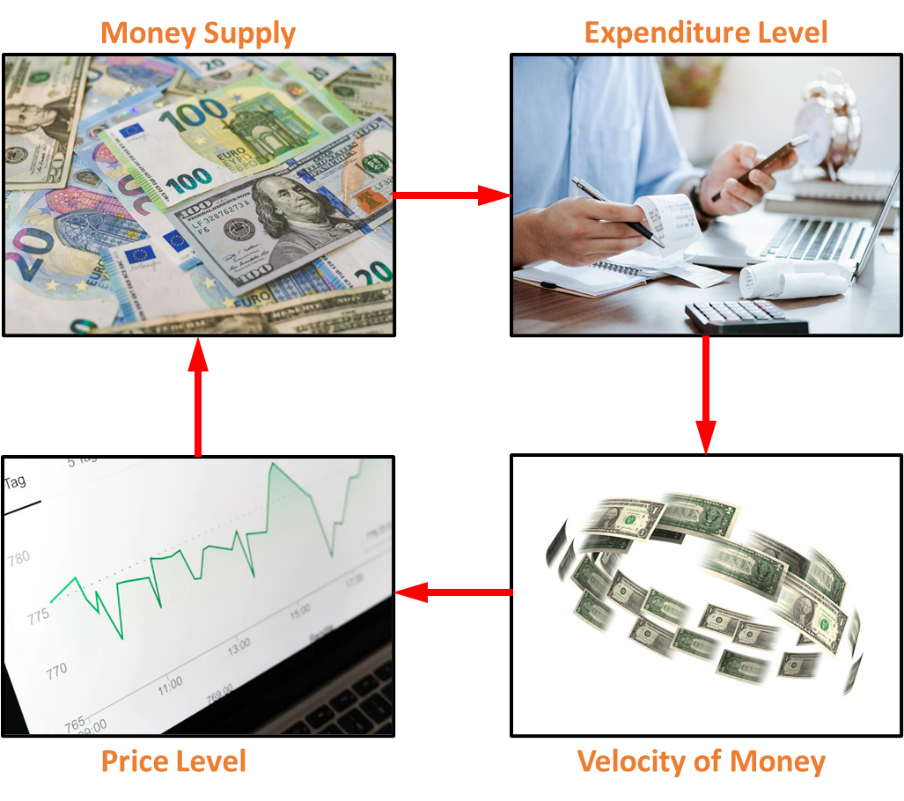In economics, this equation is a mathematical expression of the quantity theory of money. It is a mathematical expression that establishes the link or relationship between the following:

• Money Supply
• Velocity of Money
• Price Level
• Expenditure Level

Economists often refer to the monetary quantity theory of money as the equation of exchange.

This equation of exchange has also been used to argue that inflation will be proportional to changes in the money supply and that the total demand for money can be divided into two categories:

1. Demand for use in transactions
2. Demand for the sake of its liquidity.

## Background

John Stuart Mill is credited with deriving the equation for exchanging goods and services.The amount of money that changes hands in an economy is always equal to the total monetary value of goods and services that are traded means that the amount of nominal spending in an economy will always be similar to the amount of little income in an economy.

The equation of exchange is also used to argue that inflation rates will be proportional to changes in the money supply. It states that the demand for money can be broken down into the following categories:

1. The total amount of money that is needed to carry out transactions.
2. The total amount of funds required for "holding in liquidity."

This equation can be expressed as follows:

MsxV = PxT

Where:

• Ms is a measure of money supply or the average number of currency units in circulation during a period.
• V is a measure of the velocity of money or the average number of times a currency unit changes hands within a given period.
• P represents the level of average prices during that period for goods and services.
• T is the index reflecting the actual value of all transactions within a specific period.

The product of Ms & V (i.e., Ms x V) is the total amount of money that a particular economy spends in a specific period. In contrast, the product of P & T (i.e., P x T) can be interpreted as the sum of all the money spent within one's economy within a specific time.

So, it follows from the equation that the total amount of money spent within an economy by its citizens over a specific period is always equal to the total amount of goods and services over the same period.

The equation can be rewritten as follows:

MsxV = PxQ

Where:

• Money supply (Ms) is the sum of the amount in circulation in each period.
• The velocity (V) of money measures the average number of times a currency unit is exchanged during a given period.
• P is the intermediate price level of goods and services in that period.
• Q represents the index of all actual expenditures accumulated within that period.

In economic terms, the product of P and Q (i.e., P x Q) is interpreted as a measure of the nominal GDP over a period.

It is evident from the rearranged equation that the total amount of money spent within an economy over a particular period is always equal to the total amount earned during the same period; in other words, nominal expenditures are always the same as little income.

The quantity theory of money is the most explicit expression in which changes in the money supply are explained because of changes in the level of prices for goods and services.

## Quantity Theory of Money

The quantity theory of money explains how the level of prices and the level of money supply are intertwined. Irving Fisher derived this from an equation from related theoretical studies.The original theory of new quantity states that there will always be a fixed proportional relationship between changes in the money supply and the levels of prices.

To determine the relationship between the money supply and the price level, one must assume that the velocity of money and actual output are constant. This will then help determine that any change in money supply will mirror a proportional change in the price level.

To demonstrate this, first solve for P: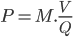Differentiating concerning time: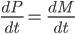This means that the inflation rate will rise proportionately with an increase in the money supply. In this way, we see the fundamental ideas behind monetarism and the inspiration behind Milton Friedman's famous assertion that "Inflation is always and everywhere - a monetary phenomenon."

The theory supports the idea that any increase in the amount of money in circulation will incite inflation, and an increase in inflation will, in turn, necessitate more cash in circulation. Let us consider the following:

Suppose the Federal Reserve prints excess money such that the amount in the economy is doubled. As more dollars purchase the same goods, there would be a dramatic price rise, resulting in more US U.S.llars being spent.

The spending and the demand for goods will likely increase in the coming period, putting upward pressure on prices.

### Quantity Theory of Money in Practice

The "Covid-19" pandemic affected the entire world in 2020. The global economy shut down, and whole populations were quarantined at home to slow down the spread of the disease.

Central banks and government organizations released a record amount of stimulus into the economy, significantly increasing the total amount of money in circulation. This stimulus aimed to prevent most people from falling into poverty and stop their incomes from reaching zero.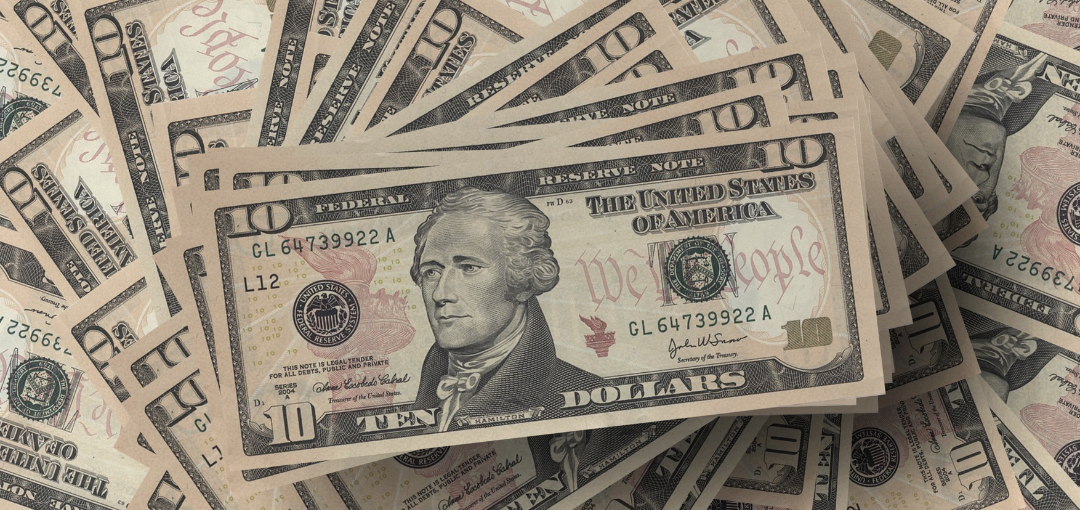Despite this, it did not translate into an increase in prices proportional to the rise in the money supply since the quantity theory of money assumes that an increase in money supply will lead directly to higher spending.

In such a situation, the money injected into the economy will not be immediately spent but instead used to pay bills and be saved. As a result of this condition, the quantity theory of money did not hold.

### Money DemandThe equation of exchange can also be used to derive the total demand for money in an economy by solving the following formula: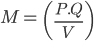If money supply equals money demand (i.e., if financial markets are in equilibrium), then we can conclude: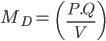Or: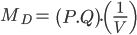According to this equation, the demand for money is proportional to the nominal income and inversely proportional to the velocity of money.

Economists usually interpret the inverse of the velocity of money as a demand to have cash on hand.

Therefore, in this version of the exchange equation, there is demand for money in an economy in terms of both transaction demand (P x Q) and liquidity demand (1/V).

## Applications: How is the Equation of Exchange Used?

Two significant benefits can be derived from the equation of exchange. The quantity theory of money is based on the principle that an increase in overall prices will occur if the money supply increases and vice versa.1. If M is calculated as per the equation, we can gain insights into the demand for money by analyzing M's value.
2. Additionally, the equation for the exchange rate can be used to provide information about the variables included in the equation. This can help in understanding the state of the economy.

Using this equation, economists can determine the impact of changes in the money supply on goods' prices. A nation's central bank is responsible for formulating the money supply and deciding whether these variables should be increased or decreased.

There are several economic theories on which monetarist approaches are based, all of which claim that the relationship between the price of goods and the money supply is an accurate indicator of the strength of an economy.

The influence that money supply creates on interest rates contrasts with the Keynesian view, which states that the economic condition of a nation determines its payroll levels. The impact payroll causes this has on interest rates and the level of employment.

### Economic Forecasts

According to the equation, if the index of expenditure and velocity of money, which are the money supply variables, remain constant over time, then an increase in the money supply would increase average price levels and vice versa.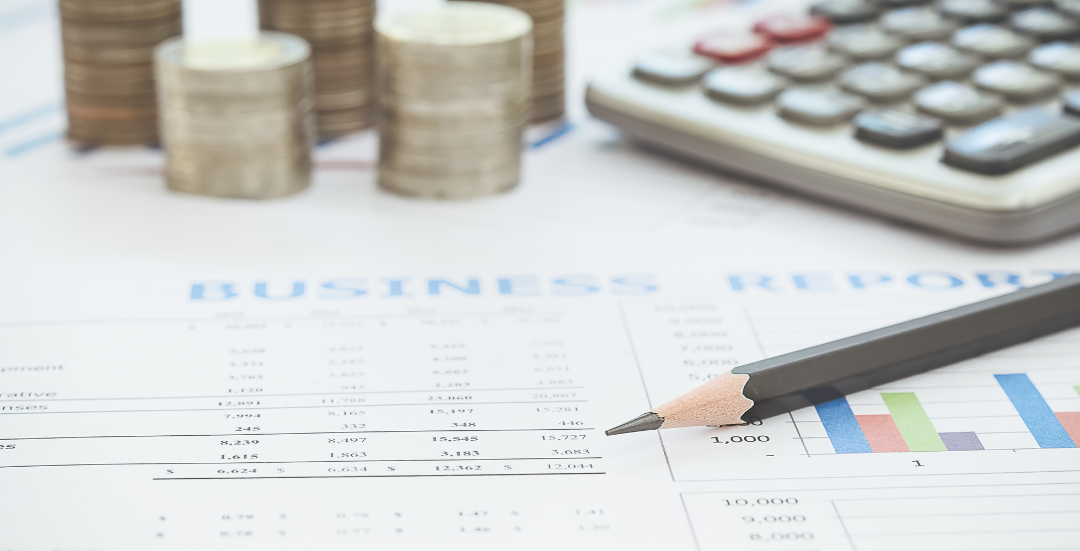Thus, it can be inferred that money supply and average price levels are directly related. It's common for Y, Q, and other letters to be used for the variable T whenever it appears in an equation.

One of the factors that influence the value of this variable is the industrial production that is connected to the final output of an economy. It is also possible to calculate the average price level of goods by dividing the gross domestic product by the actual domestic product.

Nominal GDP can be calculated by multiplying the money supply by the velocity of money. There may be cases when the variables in the equation of exchange fall regardless of what the equation predicts. This can be because of weak conditions or a country's economic situation.

### Inflation and Money Supply

The concept of inflation is an important one in economic analysis because it has a direct impact on real life. Simply put, inflation refers to an increase in price levels across the economy.An economy can suffer severe economic damage if there is a high inflation rate.

For instance, if wages don't keep up with prices, people won't be able to buy as much. To stay in business, firms must produce fewer goods and services if people can't afford to buy as much.

The frequency with which customers use the products they have already purchased will determine whether they will repeat their purchase.

There will be fewer jobs, businesses will close, and personal and business debt will not be paid if production slows down.

Several factors, such as the general level of aggregate supply and demand in the overall economy, contribute to inflation.A money supply is the total amount of money available in an economy at any given time.

Whenever you hear the term "money supply," remember that it includes the bills and coins you carry in your pocket, the electronic balance inside your savings accounts, and many other cash equivalents.

A simple way to analyze the effect of the money supply on inflation is to use the equation of exchange.

## Monetarism

The monetarist school of thought believes that the money supply (the total amount of money in an economy, in the form of coins, currency, and bank deposits) is the principal determinant of short-run economic activity.One of monetarism's most prominent proponents is American economist Milton Friedman. However, macroeconomics theorists, Friedman, and other monetarists diverge considerably from the formerly dominant Keynesian school.

The monetarist approach became dominant during the 1970s and early 1980s.

The monetarist theory relies on the equation of exchange, which is written as MV = PQ.P.Q

M represents the supply of money, V means the velocity of the deal of money (i.e., how often the average dollar in the money supply is spent on goods or services), and P represents the average price at which each good or service is sold. Q represents the number of goods produced.

According to monetarists, the money supply increases through a constant and predictable V, leading to an increase in either P or Q.

As Q increases, P will remain relatively constant. P will increase if the quantity of goods and services produced does not grow correspondingly. Production, employment, and prices are directly affected by changes in the money supply.

However, changes in the money supply become apparent once a significant period has passed. Monetary history advocates a "monetary rule" over fiscal policy.

Friedman presented a detailed analysis of the U.SU.S.ney supply from the end of the Civil War to 1960 in "A Monetary History of the United States 1867-1960 (1963)" with Anna J. Schwartz

As a result of this detailed study, other economists began to take monetarism seriously.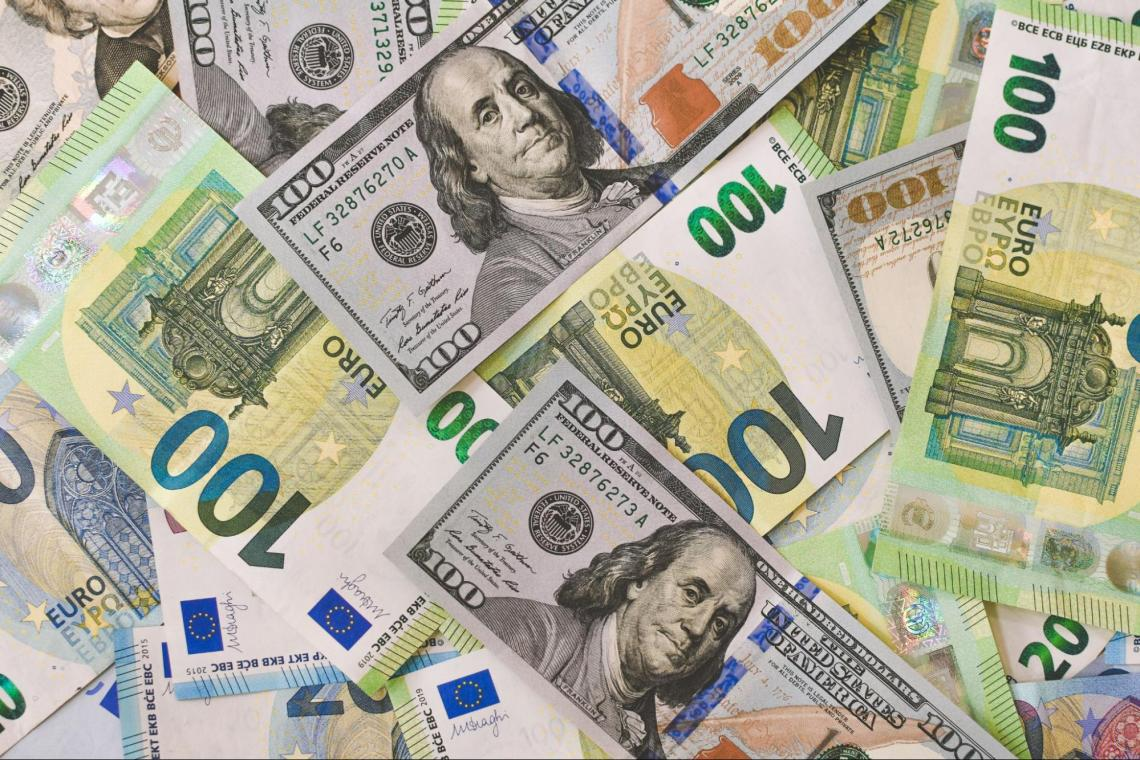Friedman argued that the government must control the money supply growth rate to promote economic stability.

This could be accomplished by following a simple rule that stipulates an increase in the money supply at a constant annual rate, tied to the potential growth of gross domestic product (GDP) (for example, an increase from 3 to 5 percent).

Thus, according to monetarism, steady, moderate money supply growth could ensure a constant economic growth rate with low inflation.

During the 1970s, monetarists maintained that inflation was a purely monetary phenomenon resulting from an increase in the money supply that outpaced growth in capacity output.

Consequently, at any given moment, inflation was seen as a reflection of current and past monetary expansion.

In the 1980s, however, changes in the U.SU.S.onomy proved that monetarism's link between economic growth and the money supply was incorrect.

• First, new and hybrid bank deposits obscured the kinds of savings traditionally used by economists to calculate the money supply.
• In addition, a decrease in inflation caused people to spend less, reducing velocity-consequently, the ability to predict the impact of money growth on nominal GDP growth diminished.### Everything You Need To Master Financial Modeling

To Help You Thrive in the Most Prestigious Jobs on Wall Street.

Researched and authored by Saif Ali | LinkedIn

Reviewed and edited by James Fazeeli-Sinaki LinkedIn